Question

Find the equivalent resistance between points A and B in the drawing.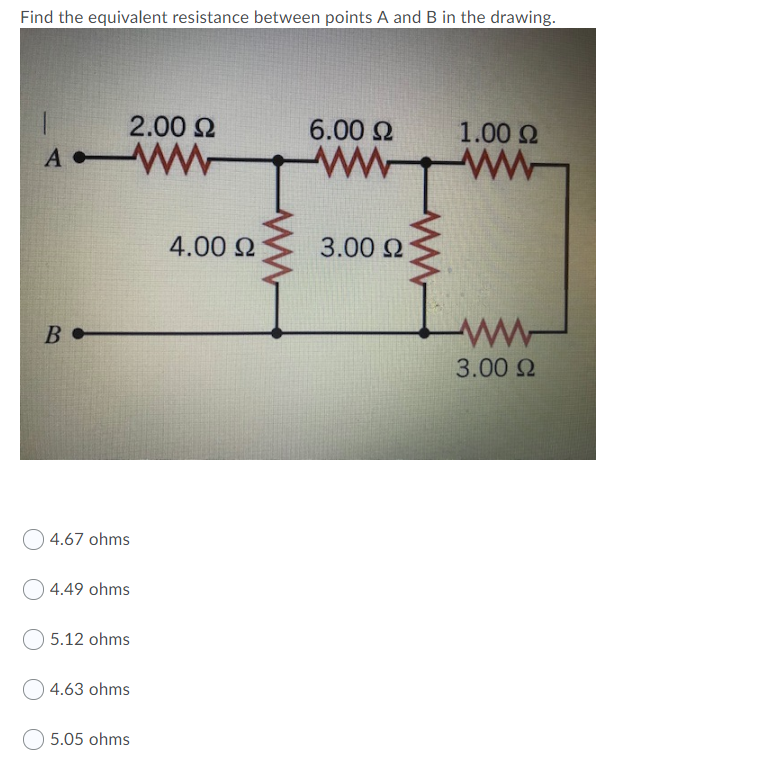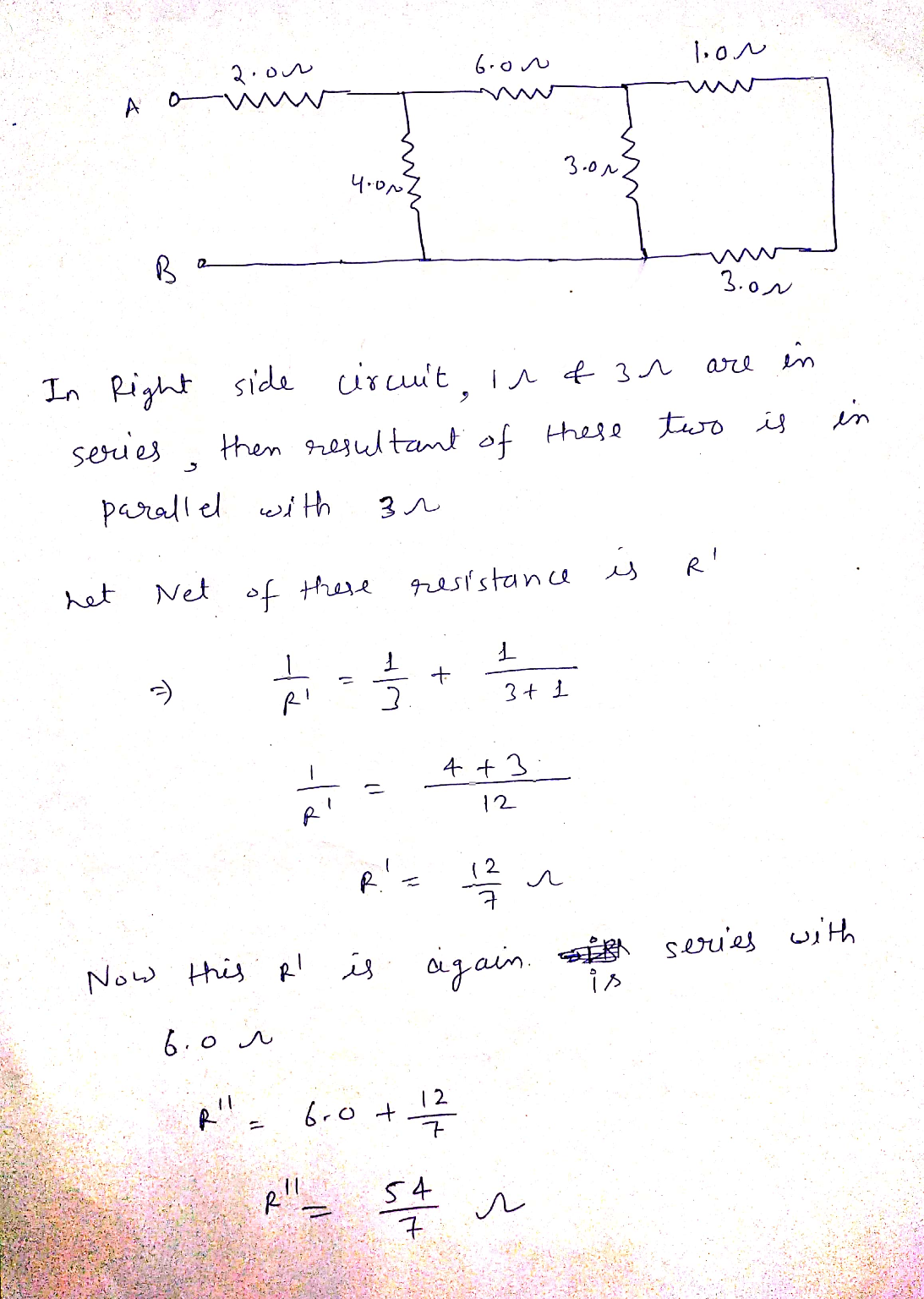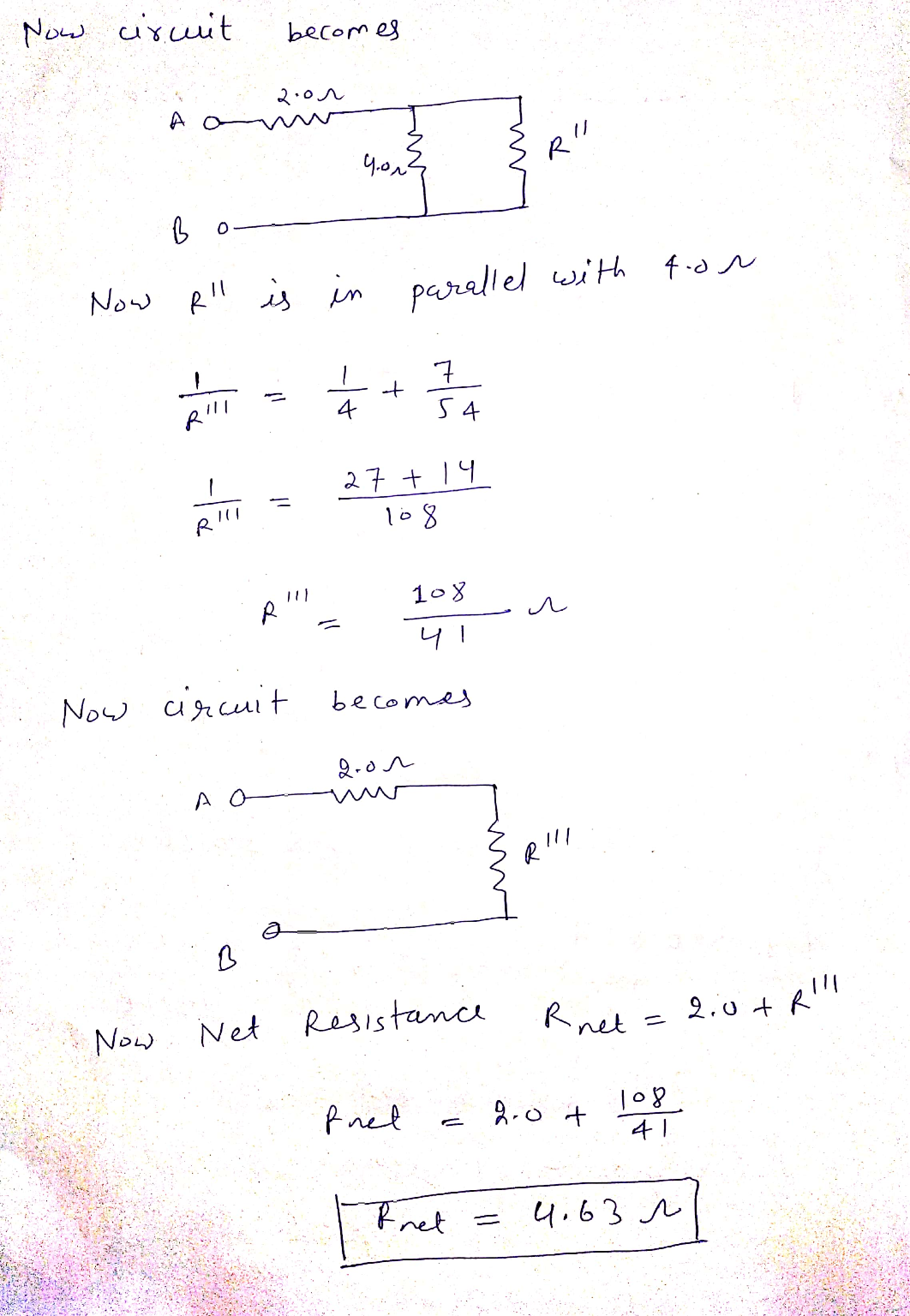#### Earn Coins

Coins can be redeemed for fabulous gifts.

Similar Homework Help Questions
• ### Find the equivalent resistance between points A and B in the drawing 2.00 Ω 6.00 Ω...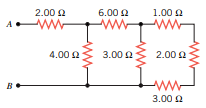Find the equivalent resistance between points A and B in the drawing 2.00 Ω 6.00 Ω 1.00 Ω wongan 4.00 Ω 3.00Ω 2.00 Ω B 3.00 Ω

• ### Find the equivalent resistance between points A and B in the drawing 2.90 Ω 6.50 Ω...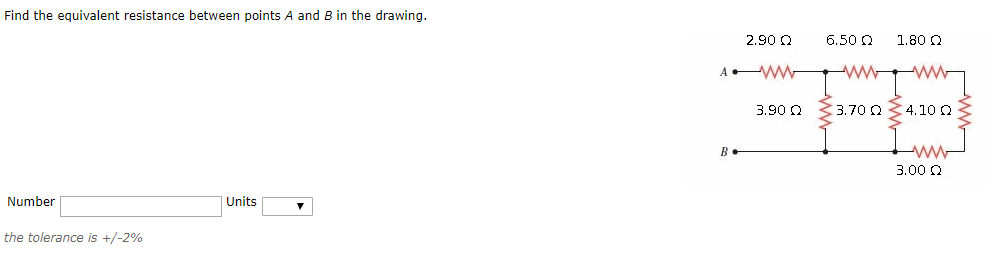Find the equivalent resistance between points A and B in the drawing 2.90 Ω 6.50 Ω 1.80 Ω 3.90 Ω 3.70 Ω 4.10 Ω 3.00 Ω Number Units the tolerance is +/-296

• ### O 0/2 points 1 Previous Answers CJ10 20.P.064 Find the equivalent resistance between points A and...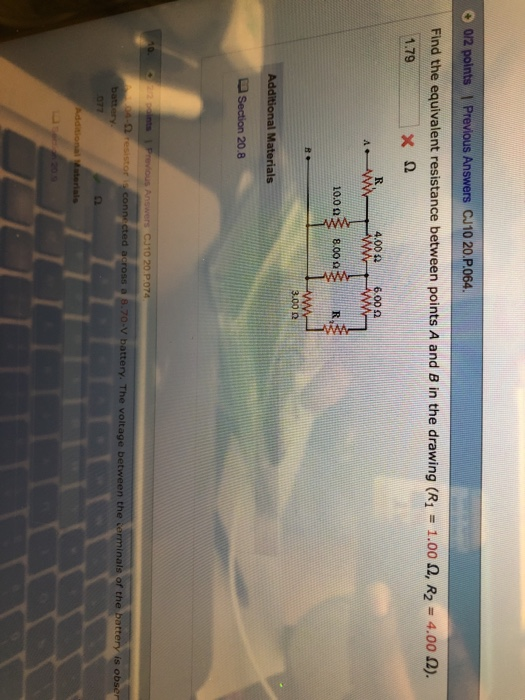O 0/2 points 1 Previous Answers CJ10 20.P.064 Find the equivalent resistance between points A and B in the drawing (R1 1.00 Ω, R2° 4.00Q) 1.79 ΧΩ 4.00 Ω 6.00 Ω 10.003 8.00 9 300 9 L Section 20.8 CJ10 20 P074 cted across a 8.70-V battery. The voltage between the cerminals of the battery is obse

• ### Find the equivalent resistance between points A and B in the drawing. 3.0 Ω 4.0 2...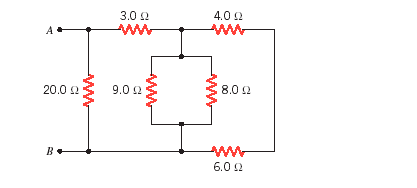Find the equivalent resistance between points A and B in the drawing. 3.0 Ω 4.0 2 8.0 Ω 20012 9.0 12 6.0 Ω

• ### Find the equivalent resistance between points A and B in the drawing. RI 26 Ω R2...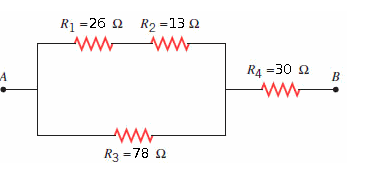Find the equivalent resistance between points A and B in the drawing. RI 26 Ω R2 1362 R4-30 Ω Ra 78 Ω

• ### 4. Find the equivalent resistance between points A and B in the drawing (R1 = 4.0...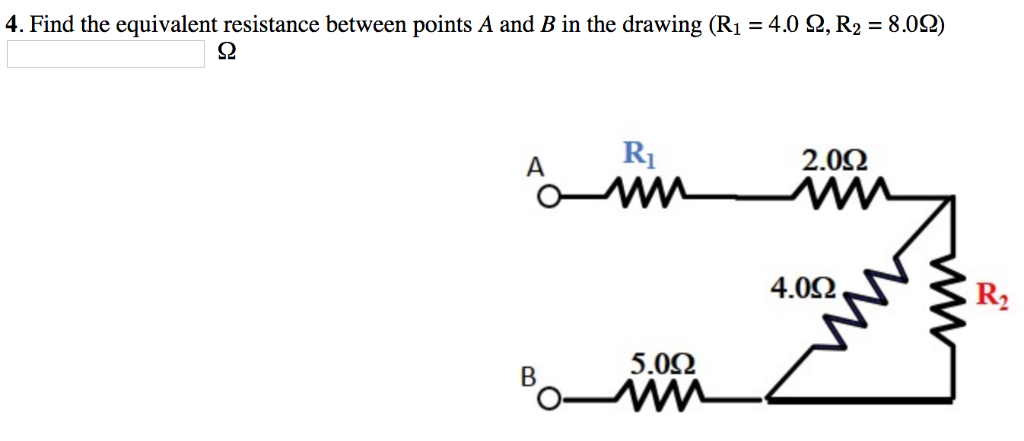4. Find the equivalent resistance between points A and B in the drawing (R1 = 4.0 Ω, R2 = 8.0Ω) S2 2.0Ω 4.0Ω R2 5.0Ω

• ### (a) Find the equivalent resistance between points a and b in the figure. (R = 17.0...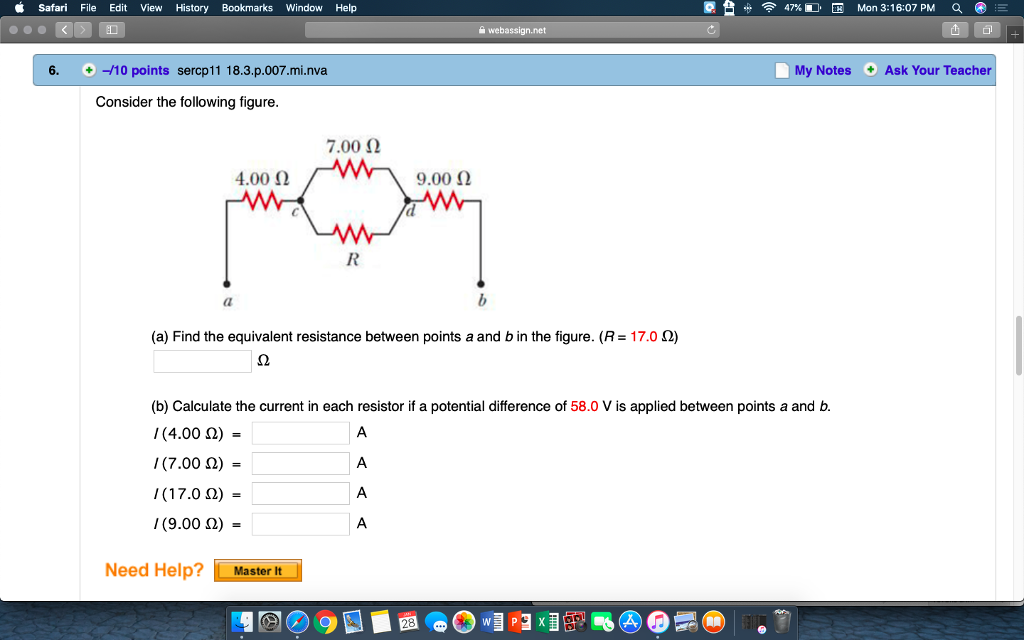(a) Find the equivalent resistance between points a and b in the figure. (R = 17.0 Ω) (b) Calculate the current in each resistor if a potential difference of 58.0 V is applied between points a and b. I (4.00 Ω) =   A I (7.00 Ω) =   A I (17.0 Ω) =   A I (9.00 Ω) =   A Please answer all parts to A and B correctly!!!! Thank you so much in advance! Safari File Edit View History Bookmarks Window...

• ### (a) Find the equivalent resistance between point a and b. (b) If a voltage of 62...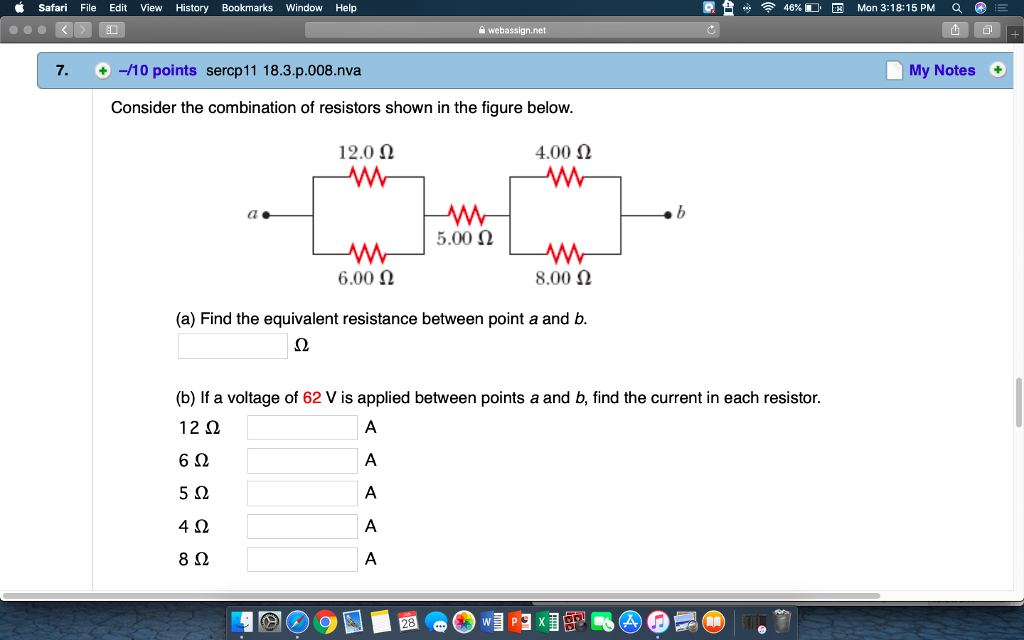(a) Find the equivalent resistance between point a and b. (b) If a voltage of 62 V is applied between points a and b, find the current in each resistor. Please answer all parts to A and B correctly!!!! Thank you so much in advance! Safari File Edit View History Bookmarks Window Help 46% Mon 3:18:15PM 'Q 7. +-/10 points sercp11 18.3.p.008.nva My Notes Consider the combination of resistors shown in the figure below. 12.0 Ω 4.00 Ω ao AM...

• ### Find the equivalent resistance between points A and B in the drawing. Number____ Units____

Find the equivalent resistance between points A and B in the drawing. Number____ Units____